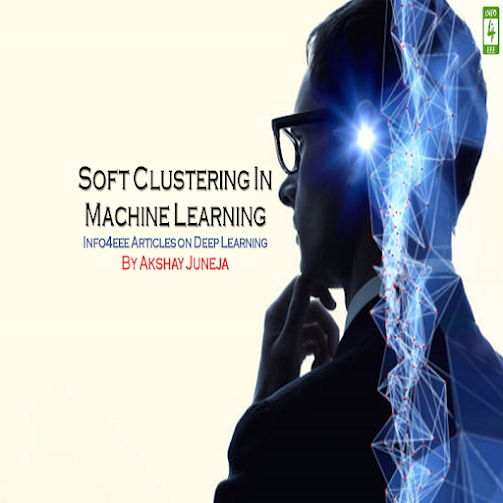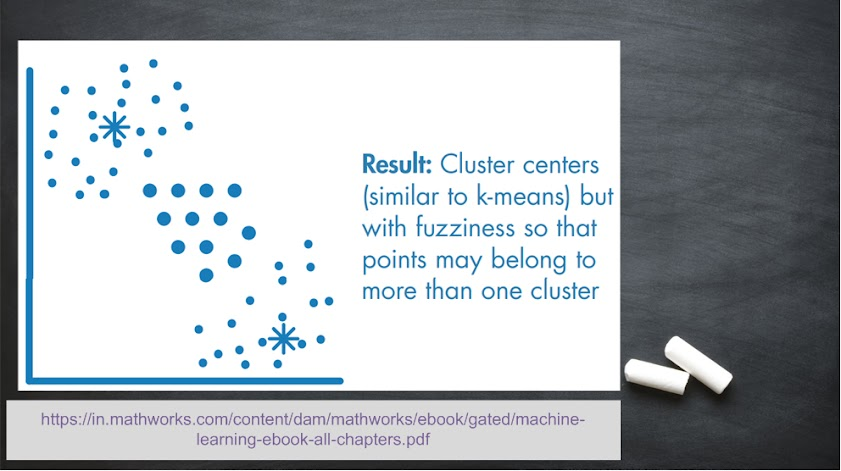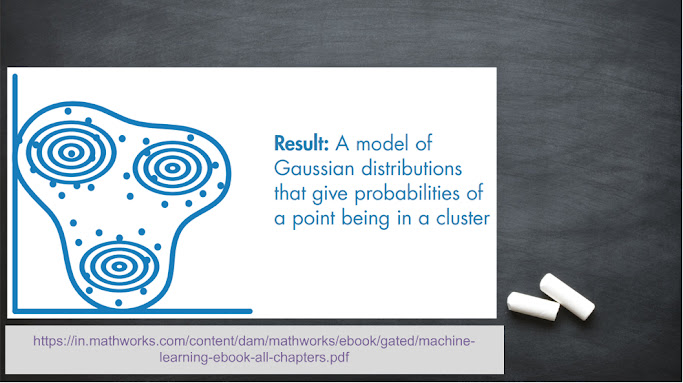Welcome back readers!! In the previous article, we discussed various hard clustering techniques. In this article, we will discuss soft clustering techniques. They are categorized as Fuzzy c-Means and Gaussian Mixture Model.

#### Fuzzy c-Means

The concept of fuzzy logic comes into play when the probability of occurrence of an event can be any value between 0 and 1. So, there are possibilities that a data value can belong to more than one cluster. The algorithm is designed similar to k-Means clustering, but centres are estimated using fuzzy logic. Key points are as follows:

• Used when number of clusters is known.
• Used for pattern recognition.
• Used when clusters overlap.#### Gaussian Mixture Model

When there are different multivariate Gaussian or Normal distributions of data points, each distribution with a certain probability, Gaussian Mixture Model is used. Key points are as follows:

• Used when the data point belongs to more than one cluster.
• Used when clusters have different sizes and correlation structures within them.Reference: Machine Learning with MATLAB (eBook)